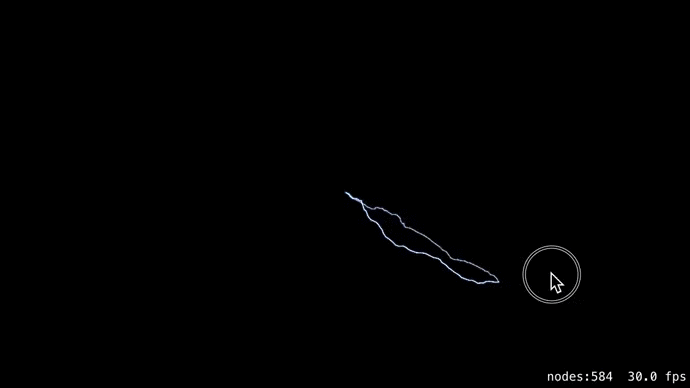Have you ever tried to implement such effect as lightning for your iOS game? In this article I’m going to describe three ways I found to create a lightning effect with a decent quality:

• SKShapeNode.
• CAShapeLayer.
• SKSpriteNodes.

For each of them we need to compose a path for our lightning bolt. I searched the web and found this article about procedural generation of lightning bolt. This approach uses Midpoint Displacement algorithm. The result looks pretty realistic. Here is Objective-C code for Midpoint Displacement algorithm:

``````void createBolt(float x1, float y1, float x2, float y2, float displace, UIBezierPath *path) {
if (displace < 1.8f) {
CGPoint point = CGPointMake(x2, y2);
[path MoveToPoint:point];
}
else {
float mid_x = (x2+x1)*0.5f;
float mid_y = (y2+y1)*0.5f;
mid_x += (arc4random_uniform(100)*0.01f-0.5f)*displace;
mid_y += (arc4random_uniform(100)*0.01f-0.5f)*displace;
createBolt(x1, y1, mid_x, mid_y, displace*0.5f, path);
createBolt(mid_x, mid_y, x2, y2, displace*0.5f, path);
}
}
``````

Ok, we got the path, how about drawing it? “We can define `SKShapeNode` with our path!” — that’s how I thought.

### SKShapeNode.

Let’s create `SKShapeNode` with `UIBezierPath`, created from our pathArray:

``````- (void)addBoltWithStartPoint:(CGPoint)startPoint endPoint:(CGPoint)endPoint {
// Dynamically calculating displace
// Distance between two points
float hypot = hypotf(fabsf(endPoint.x - startPoint.x), fabsf(endPoint.y - startPoint.y));
// hypot/displace = 4/1
float displace = hypot*0.25;

UIBezierPath *path = [UIBezierPath bezierPath];
[path moveToPoint:startPoint];

createBoltPath(startPoint.x, startPoint.y, endPoint.x, endPoint.y, displace, path);

SKShapeNode *bolt = [SKShapeNode node];
bolt.path = path.CGPath;
bolt.strokeColor = [SKColor whiteColor];
bolt.lineWidth = 0.5f;
bolt.antialiased = NO;

SKShapeNode *shadowNode = [[SKShapeNode alloc] init];
shadowNode.strokeColor = [SKColor colorWithRed:0.702 green:0.745 blue:1 alpha:1.0];
}

- (void)createBoltWithPath:(UIBezierPath*)path {
BoltNode *bolt = [[BoltNode alloc] initWithBezierPath:path lifetime:0.5f];
}
``````

Defining `SKShapeNode` with `UIBezierPath` seems very simple, and result looks nice and realistic, but if you use a lot of such nodes, FPS dramatically drops. That didn’t suit me, so I came to the second approach: using CAShapeLayer.

### CAShapeLayer.

`CAShapeLayer` allows us to draw a path on our view’s `CALayer`. Moreover, it offers a shadow setting for the path out of the box: by using shadowColor, shadowOffset, shadowRadius and shadowOpacity properties. Ok, let’s try that!

``````- (void)addBoltWithStartPoint:(CGPoint)startPoint endPoint:(CGPoint)endPoint {
// Dynamically calculating displace
// Distance between two points
float hypot = hypotf(fabsf(endPoint.x - startPoint.x), fabsf(endPoint.y - startPoint.y));
// hypot/displace = 4/1
float displace = hypot*0.25;

UIBezierPath *path = [UIBezierPath bezierPath];
[path moveToPoint:startPoint];

createBoltPath(startPoint.x, startPoint.y, endPoint.x, endPoint.y, displace, path);

CAShapeLayer *shapeLayer = [CAShapeLayer layer];
shapeLayer.path = bezierPath.CGPath;
shapeLayer.strokeColor = [[UIColor whiteColor] CGColor];
shapeLayer.lineWidth = 1.f;
shapeLayer.fillColor = [UIColor clearColor].CGColor;
shapeLayer.zPosition = 20;
shapeLayer.shadowColor = [UIColor colorWithRed:0.702 green:0.745 blue:1 alpha:1.0].CGColor;
shapeLayer.shouldRasterize = YES;
}
``````

Using `CAShapeLayer` seems much more efficient than `SKShapeNode`:

But there are several cons:

• The shadow looks very pale and unnatural
• You cannot change zPosition of shapeLayer on `SKScene`. Thus you cannot place any of your `SKNodes` above shapeLayer.

I googled about other methods of lightning generation and found a great article “How to Generate Shockingly Good 2D Lightning Effects” by Michael Hoffman. He has written his project using C# and XNA, so I decided to make the same trick with using Objective-C and Sprite Kit’s `SKSpriteNode`!

### SKSpriteNode.

First, we need to draw our building blocks: a half-circle image and a lightning segment. Here is what I got so far:

Second, Michael uses his own method to generate a lightning bolt path, but Midpoint Displacement algorithm seems more realistic for me, so I decided to keep using it. We don’t need `UIBezierPath` with this approach, so let’s slightly change the code of createBolt function:

``````void createBolt(float x1, float y1, float x2, float y2, float displace, NSMutableArray *pathArray) {
if (displace < 1.8f) {
CGPoint point = CGPointMake(x2, y2);
}
else {
float mid_x = (x2+x1)*0.5f;
float mid_y = (y2+y1)*0.5f;
mid_x += (arc4random_uniform(100)*0.01f-0.5f)*displace;
mid_y += (arc4random_uniform(100)*0.01f-0.5f)*displace;
createBolt(x1, y1, mid_x, mid_y, displace*0.5f, pathArray);
createBolt(mid_x, mid_y, x2, y2, displace*0.5f, pathArray);
}
}
``````

Third, let’s create a special `SKNode` called LightningLine, which represents one single line of our path:

``````@interface LightningLine : SKNode

@property (nonatomic) CGPoint startPoint;
@property (nonatomic) CGPoint endPoint;
@property (nonatomic) float thickness;

- (instancetype)initWithStartPoint:(CGPoint)startPoint endPoint:(CGPoint)endPoint;
- (void)draw;

@end

@implementation LightningLine

- (instancetype)initWithStartPoint:(CGPoint)startPoint endPoint:(CGPoint)endPoint {
if (self = [super init]) {
self.startPoint = startPoint;
self.endPoint = endPoint;
self.thickness = 1.3f;
}
return self;
}

- (void)draw {
const float imageThickness = 2.f;
float thicknessScale = self.thickness / imageThickness;
CGPoint startPointInThisNode = [self convertPoint:self.startPoint fromNode:self.parent];
CGPoint endPointInThisNode = [self convertPoint:self.endPoint fromNode:self.parent];
float angle = atan2(endPointInThisNode.y - startPointInThisNode.y,
endPointInThisNode.x - startPointInThisNode.x);
float length = hypotf(fabsf(endPointInThisNode.x - startPointInThisNode.x),
fabsf(endPointInThisNode.y - startPointInThisNode.y));

SKSpriteNode *halfCircleA = [SKSpriteNode spriteNodeWithTexture:[self halfCircle]];
halfCircleA.anchorPoint = CGPointMake(1, 0.5);
SKSpriteNode *halfCircleB = [SKSpriteNode spriteNodeWithTexture:[self halfCircle]];
halfCircleB.anchorPoint = CGPointMake(1, 0.5);
halfCircleB.xScale = -1.f;
SKSpriteNode *lightningSegment = [SKSpriteNode spriteNodeWithTexture:[self lightningSegment]];
halfCircleA.yScale = halfCircleB.yScale = lightningSegment.yScale = thicknessScale;
halfCircleA.zRotation = halfCircleB.zRotation = lightningSegment.zRotation = angle;
lightningSegment.xScale = length*2;

halfCircleA.blendMode = halfCircleB.blendMode = lightningSegment.blendMode = SKBlendModeAlpha;

halfCircleA.position = startPointInThisNode;
halfCircleB.position = endPointInThisNode;
lightningSegment.position = CGPointMake((startPointInThisNode.x + endPointInThisNode.x)*0.5f,
(startPointInThisNode.y + endPointInThisNode.y)*0.5f);
}

static dispatch_once_t onceToken;
dispatch_once(&onceToken, ^{
sHalfCircle = [SKTexture textureWithImageNamed:@"half_circle"];
sLightningSegment = [SKTexture textureWithImageNamed:@"lightning_segment"];
});
}

static SKTexture *sHalfCircle = nil;
- (SKTexture*)halfCircle {
return sHalfCircle;
}

static SKTexture *sLightningSegment = nil;
- (SKTexture*)lightningSegment {
return sLightningSegment;
}

@end
``````

loadSharedAssets method loads our images to static variables, so it must be called before we initialize a node. The best way to call it is `SKScene`’s init method.

``````- (instancetype)initWithSize:(CGSize)size {
if (self = [super initWithSize:size]) {
}
return self;
}
``````

Finally, here is a method, that does all magic:

``````- (void)drawBoltFromPoint:(CGPoint)startPoint toPoint:(CGPoint)endPoint {
// Dynamically calculating displace
float hypot = hypotf(fabsf(endPoint.x - startPoint.x), fabsf(endPoint.y - startPoint.y));
// hypot/displace = 4/1
float displace = hypot*0.25;
float angle = atan2(endPoint.x - startPoint.x, endPoint.y - startPoint.y);

NSMutableArray *pathArray = [NSMutableArray array];
createBolt(startPoint.x, startPoint.y, endPoint.x, endPoint.y, displace, pathArray);
//    NSMutableArray *boltLines = [NSMutableArray array];
for (int i = 0; i < pathArray.count - 1; i = i + 1) {
LightningLine *line = [[LightningLine alloc] initWithStartPoint:((NSValue *)pathArray[i]).CGPointValue
endPoint:((NSValue *)pathArray[i+1]).CGPointValue];